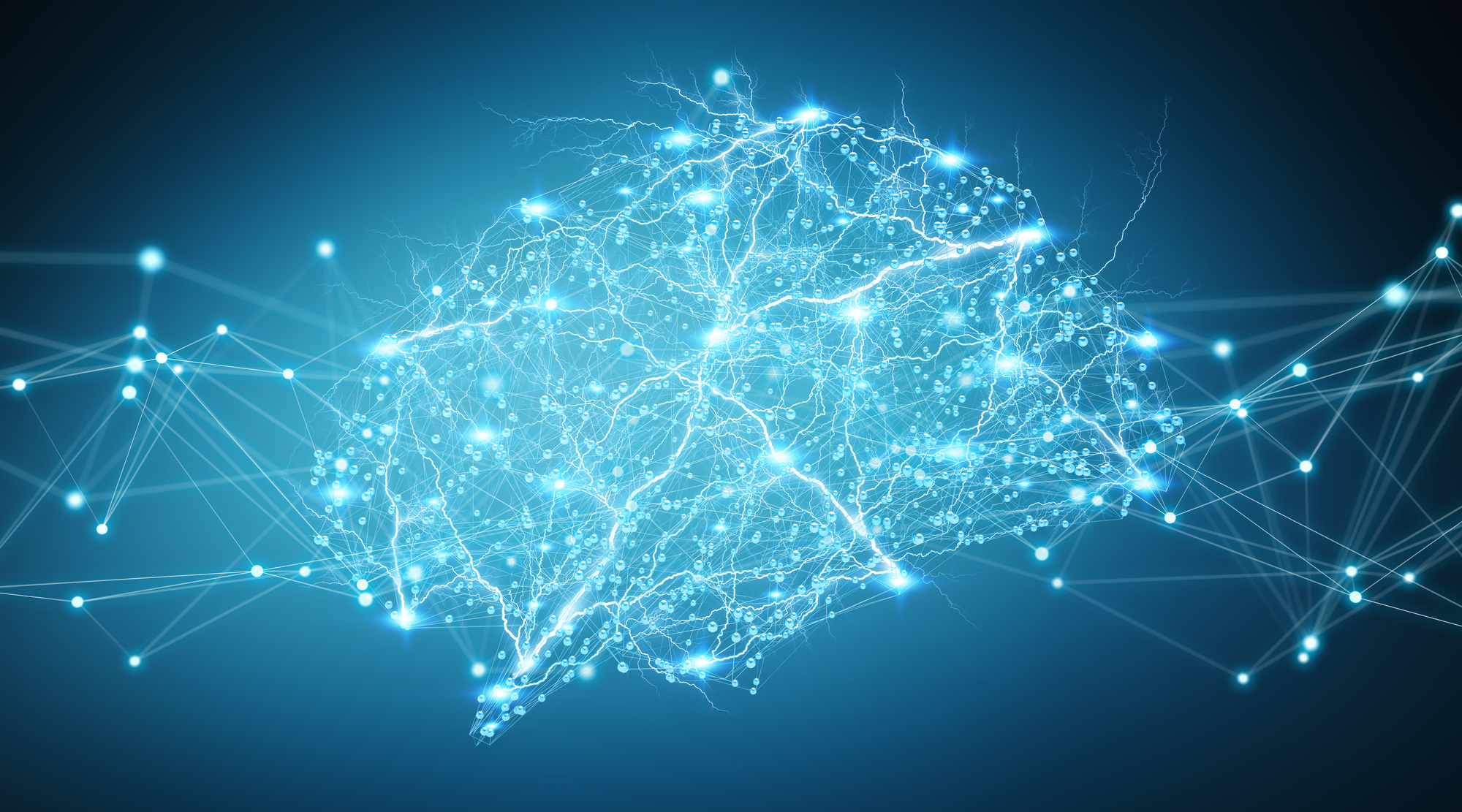## Deep Learning Interview Questions

Why Deep Learning is better than Machine Learning?

Machine Learning algorithms are not useful while working with high dimensional data, that is where we have a large number of inputs and outputs. For example, in the case of handwriting recognition, we have a large amount of input where we will have a different type of inputs associated with different type of handwriting. And the major challenge is to tell the computer what are the features it should look for that will play an important role in predicting the outcome as well as to achieve better accuracy while doing so.

What is the significance of a loss function?

A loss function is a measure of the accuracy of the neural network with respect to a given training sample and expected output. It provides the performance of a neural network as a whole. In deep learning, the goal is to minimize the cost function. For that, we use the concept of gradient descent.

Gradient descent is an optimization algorithm used to minimize some function by iteratively moving in the direction of steepest descent as defined by the negative of the gradient.

What is backpropagation?

Backpropagation is a method to update the weights in the neural network by taking into account the actual output and the desired output. It is a training algorithm used for a multilayer neural network. It moves the error information from the end of the network to all the weights inside the network and thus allows for efficient computation of the gradient.

What are some of the advantages of CNN over a fully connected neural network?

The main advantage of CNN compared to its predecessors is that it automatically detects the important features without any human supervision. CNN is also computationally efficient. It uses special convolution and pooling operations and performs parameter sharing. This enables CNN models to run on any device, making them universally attractive.

What is Dropout & Batch Normalization? Why are they used?

Dropout is a cheap regulation technique used for reducing overfitting in neural networks. We randomly drop out a set of nodes at each training step. As a result, we create a different model for each training case, and all of these models share weights. It’s a form of model averaging.

Batch normalization is a technique to standardize the inputs to a network, applied to ether the activations of a prior layer or inputs directly. It is a technique for training very deep neural networks that standardizes the inputs to a layer for each mini-batch. This has the effect of stabilizing the learning process and dramatically reducing the number of training epochs required to train deep networks

How does an LSTM model work?

LSTMs, are special kind of Recurrent Neural Networks that are capable of learning long-term dependencies i.e. remembering the information for a longer period of time is their default behavior.

There are 3 steps in the process:

- Decides what to forget and what to remember

- Selectively updates the cell state values

- Decides what part of the current state make it to the output